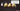# Three Sum Closest - Leet Code Solution

September 13, 2019## Problem Statement

Given an array nums of n integers and an integer target, find three integers in nums such that the sum is closest to target. Return the sum of the three integers. You may assume that each input would have exactly one solution.

``````Example:

Given array nums = [-1, 2, 1, -4], and target = 1.
The sum that is closest to the target is 2. (-1 + 2 + 1 = 2).``````

## Solution-1

The obvious brute force algorithm. The key is to get the closest. You need to compare

``Math.abs(target - calculated_sum)``

## Solution-2

Lets look at the optimized solution.

1. Sort the array
2. Have left and right pointers.
3. Keep calculating sum of three values.
4. Compare absolute value of difference between targetSum and calculated sum.
5. If sum is lesser, need to move left pointer
6. else move right pointer.
``````public int threeSumClosest(int[] nums, int target) {
Arrays.sort(nums);

int l = nums.length;
int minDiff = Integer.MAX_VALUE;
int result = 0;
for (int i=0; i<l; i++) {
int j=i+1;
int k=l-1;

while (j < k) {
int sum = nums[i] + nums[j] + nums[k];
if (sum == target) return sum;
else if (sum < target) j++;
else k--;

if (Math.abs(target - sum) < minDiff) {
minDiff = Math.abs(target - sum);
result = sum;
}
}
}

return result;
}``````